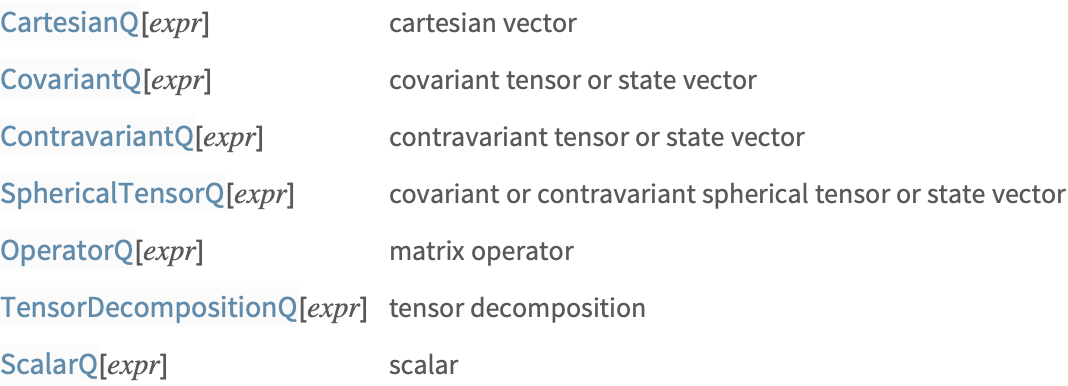# Matrix Notation

In quantum-mechanical matrix notation, the expansion coefficients of a general ket in a particular basis are notated as a column vector, expansion coefficients of a bra are notated as a row vector, and operators are notated as a square matrix. This notation is adapted for use in the AtomicDensityMatrix package.

Mathematica does not distinguish between row and column vectors. Therefore n×1 and 1×n matrices are used for this purpose. The expansion coefficients of a ket with a particular value of j in terms of the |j m basis form a contravariant irreducible tensor set. Generalizing the notation, we represent the contravariant components of any irreducible tensor in the spherical basis as a (1×n) column vector. Likewise, the covariant components of an irreducible tensor in the spherical basis are represented as a (n×1) row vector. Operators are represented by n×n square matrices.

There is one ambiguous case: A 1×1 matrix satisfies the form of a covariant or contravariant tensor, or an operator. However, since in any case the object transforms as a scalar, this does not cause much difficulty.Checking for various structures in matrix notation.

Cartesian vectors are represented as standard Mathematica vectors: one-dimensional lists.

 Here is a Cartesian vector.
 This checks for the form of a Cartesian vector.
 Here is a contravariant state vector (a 31 array). In MatrixForm it is displayed as a column vector.
 This checks if the expression is in contravariant (n×1) form.
 Here is a covariant state vector (a 13 array). In MatrixForm it is displayed as a row vector.
 This checks if the expression is in covariant (1×n) form.

Operators are represented by (n×n)-dimensional lists.

 Here is an operator.
 This checks for the (n×n) form of an operator.

The components of a Cartesian vector or spherical tensor can themselves be operators.

 Here are the spherical components of the J=1/2 representation of the angular momentum operator, which make up a covariant rank-1 tensor. MatrixForm displays it as a row vector of matrix operators.
 It has the form of a covariant tensor.
 The contravariant components are obtained by taking the Hermitian conjugate. MatrixForm displays the resulting expression as a column vector of matrix operators.
 Now it has the form of a contravariant tensor.

The list of Cartesian components of a vector operator is not displayed in the proper format by MatrixForm; what we want is each component displayed as a matrix. To do this we can use the TensorForm function, which is equivalent in this case to applying MatrixForm to each component. TensorForm could also have been used in the above cases, where it would have produced the same result as MatrixForm.

 Here are the Cartesian components of the operator (proportional to the Pauli matrices), displayed using TensorForm.
 The components form a Cartesian vector.

Any operator representation on a space with angular momentum J can be decomposed into a sum of irreducible tensor operators. The resulting decomposition is represented as a list of spherical tensors with ranks zero through 2J.

 Here is the tensor decomposition of an operator on a J=1 space (e.g., the density matrix for a J=1 state). It consists of a rank-zero, rank-one and rank-two operator.
 A tensor decomposition is a list of irreducible tensors of increasing rank.

An expression is considered to be a scalar if it does not match any of the tensor forms listed above.

 Any unknown variable is considered to be a scalar.